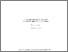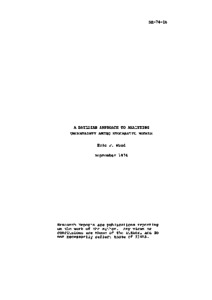# A Bayesian Approach to Analyzing Uncertainty Among Stochastic Models

Wood, E.F. (1974). A Bayesian Approach to Analyzing Uncertainty Among Stochastic Models. IIASA Research Report. IIASA, Laxenburg, Austria: RR-74-016Preview
Text
RR-74-016.pdf

## Abstract

The statistical uncertainty, resulting from the lack of knowledge of which modeling represents a given stochastic process, is analyzed. This analysis of model uncertainty leads to a composite Bayesian distribution. The composite Bayesian distribution is a linear model of the individual Bayesian probability distributions of the individual models, weighted by the posterior probability that a particular model is the true model. The composite Bayesian probability model accounts for all sources of statistical uncertainty -- both parameter uncertainty and model uncertainty. This model is the one that should be used in applied problems of decision analysis, for it best represents the knowledge -- or lack of it -- to the decision maker about future events of the process.

Item Type: Monograph (IIASA Research Report) System and Decision Sciences - Core (SDS)Resources and Environment Area (REN) IIASA Import 15 Jan 2016 01:40 27 Aug 2021 17:07 http://pure.iiasa.ac.at/78View Item